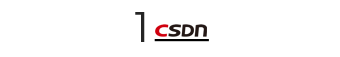### 栈的概念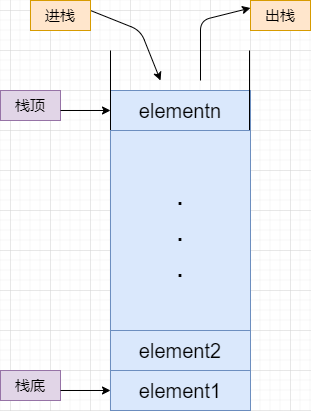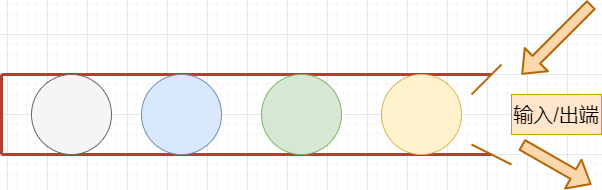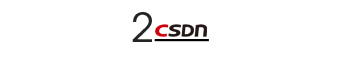### 栈的结点设计

• 数组栈使用数组进行功能的模拟，实现较为快速和便利；

• 链表栈使用链表的思路去设计，实现相比较说较为麻烦，但是其稳定，且不易出错；

• 在链表栈中又分为静态链表栈和动态链表栈，其区别如下：

• 静态链表栈给定栈的空间大小，不允许超过存储超过给定数据大小的元素；

• 动态栈使用的是自动创建空间的方法进行创建，只要符合机器的硬件要求以及编译器的控制，其理论上是极大的。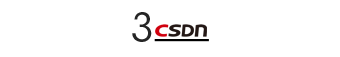#### 数组栈

1. 元素所占的存储空间必须连续，这里的连续是指的逻辑连续，而不是物理连续。

2. 元素在存储空间的位置是按逻辑顺序存放的。

``````#define STACK_INIT_SIZE 1000 //数值可以根据实际情况确定
#define STACK_INCREMENT 10   //数值可以根据实际情况确定
``````

``````typedef struct
{
void *base;
void *top;
int stackSize;
} SqSTACK;

``````
• base 表示栈底指针

• top 表示栈顶指针

• stackSize 表示栈当前可以使用的最大容量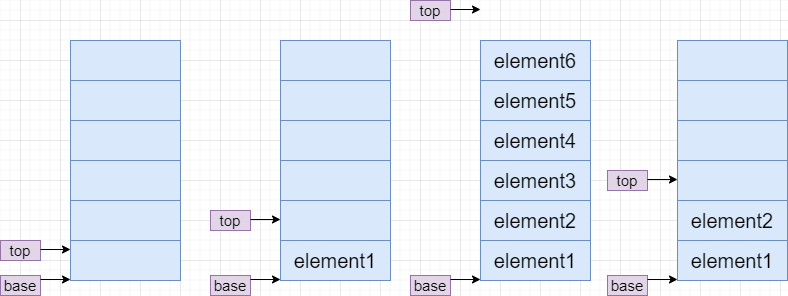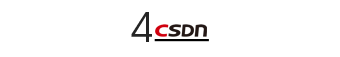NodeStack

``````//栈的结点设计
//单个结点设计，数据和下一个指针
typedef struct node
{
int data;
struct node *next;
} Node;

``````

``````typedef struct stack
{
Node *top;
int count;

``````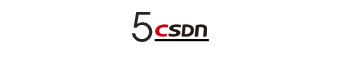### 栈的基本操作—入栈（压栈）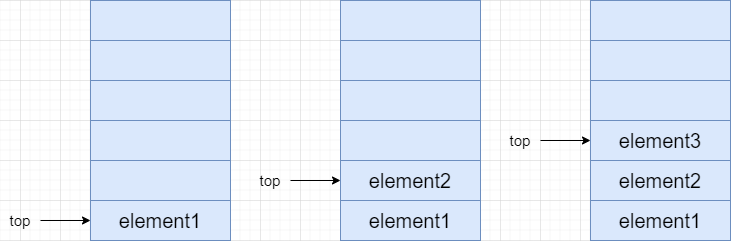``````//入栈 push
{
if (p == NULL)
return NULL;
Node *temp;
temp=(Node*)malloc(sizeof(Node));
//temp = new Node;
temp->data = elem;
temp->next = p->top;
p->top = temp;
p->count++;
return p;
}

``````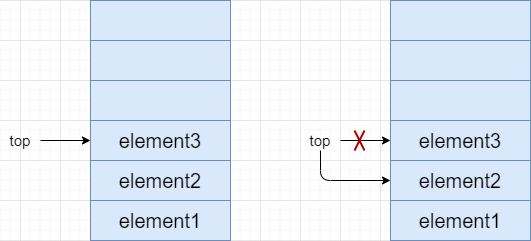``````//出栈 pop
{
Node *temp;
temp = p->top;
if (p->top == NULL)
{
printf("错误：栈为空");
return p;
}
else
{
p->top = p->top->next;
free(temp);
//delete temp;
p->count--;
return p;
}
}

``````

``````//遍历栈：输出栈中所有元素
{
Node *temp;
temp = p->top;
if (p->top == NULL)
{
printf("");
printf("错误：栈为空");
return 0;
}
while (temp != NULL)
{
printf("%d\t", temp->data);
temp = temp->next;
}
printf("\n");
return 0;
}

``````

### 栈数组与栈链表的代码实现

#### 栈数组

``````#include<stdio.h>
#include<stdlib.h>
#include<string.h>
#define maxn 10000

//结点设计
typedef struct stack{
int data[maxn];
int top;
}stack;

//创建
stack *init(){
stack *s=(stack *)malloc(sizeof(stack));
if(s==NULL){
printf("分配内存空间失败");
exit(0);
}
memset(s->data,0,sizeof(s->data));
//memset操作来自于库文件string.h，其表示将整个空间进行初始化
s->top=0;     //栈的top和bottom均为0（表示为空）
return s;
}

//入栈push
void push(stack *s,int data){
s->data[s->top]=data;
s->top++;
}

//出栈pop
void pop(stack *s){
if(s->top!=0){
s->data[s->top]=0;  //让其回归0模拟表示未初始化即可
s->top--;
}
}

//模拟打印栈中元素
void print_stack(stack *s){
for(int n=s->top-1;n>=0;n--){
printf("%d\t",s->data[n]);
}
printf("\n");   //习惯性换行
}

int main(){
stack *s=init();
int input={11,22,33,44,55};  //模拟五个输入数据
for(int i=0;i<5;i++){
push(s,input[i]);
}
print_stack(s);
/
pop(s);
print_stack(s);
return 0;
}
``````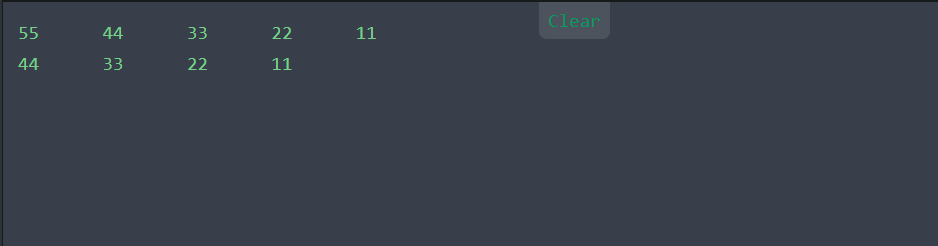#### 栈链表

``````#include <stdio.h>
#include <stdlib.h>
//栈的结点设计
//单个结点设计，数据和下一个指针
typedef struct node
{
int data;
struct node *next;
} Node;
//利用上面的结点创建栈，分为指向头结点的top指针和计数用的count
typedef struct stack
{
Node *top;
int count;

//创建栈
{
if(p==NULL){
printf("创建失败，即将退出程序");
exit(0);
}
else
{printf("创建成功\n");
}
p->count = 0;
p->top = NULL;
return p;
}

//入栈 push
{
if (p == NULL)
return NULL;
Node *temp;
temp=(Node*)malloc(sizeof(Node));
//temp = new Node;
temp->data = elem;
temp->next = p->top;
p->top = temp;
p->count++;
return p;
}

//出栈 pop
{
Node *temp;
temp = p->top;
if (p->top == NULL)
{
printf("错误：栈为空");
return p;
}
else
{
printf("\npop success");
p->top = p->top->next;
free(temp);
//delete temp;
p->count--;
return p;
}
}

//遍历栈：输出栈中所有元素
{
Node *temp;
temp = p->top;
if (p->top == NULL)
{
printf("");
printf("错误：栈为空");
return 0;
}
while (temp != NULL)
{
printf("%d\t", temp->data);
temp = temp->next;
}
printf("\n");
return 0;
}

int main()
{ //用主函数测试一下功能
int i;
p = Creat_stack();
int n = 5;
int input = {10,20,30,40,50,60};
/以依次入栈的方式创建整个栈//
for(i=0;i<n;i++){
Push_stack(p, input[i]);
}
show_stack(p);
出栈///
Pop_stack(p);
show_stack(p);
return 0;
}
``````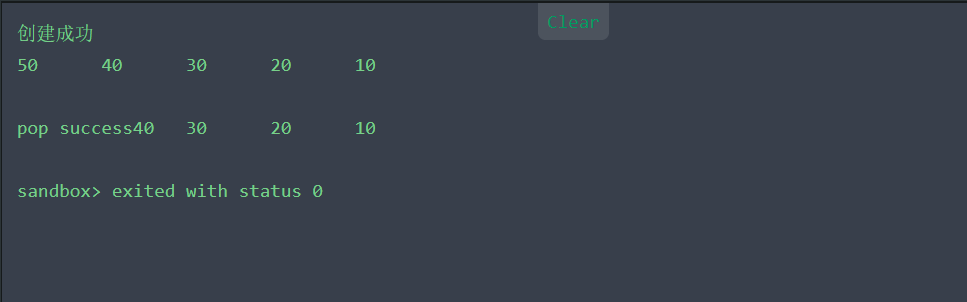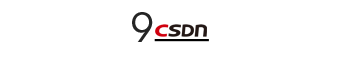### 关于栈的总结20年前，《新程序员》创刊时，我们的心愿是全面关注程序员成长，中国将拥有新一代世界级的程序员。20年后的今天，我们有了新的使命：助力中国IT技术人成长，成就一亿技术人！